# Descending number sequence with Dates (Months) as the headingAssuming my heading is the time: Jan 19, Feb 19 to Dec. 20. This is due to a parameter set. and the range changes based on the parameter to be set.

My line item will be Date in number. this will make Jan 20 - Dec 20 to 1, 2, 3 and so on. I have successfully sequenced Jan 20 - Dec 20.

However Jan 19 to Dec 19 will have to be sequenced as -12, -11, -10 and so one. Making Dec 19 the -1 in the sequence.

How can i possibly pull this off using a number format?

Thanks,

Elaine

Tagged:

•Absolutely!

Here's my time selection module and formulas: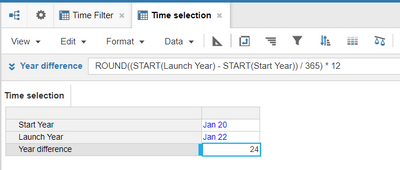The Year difference formula is very important:

`ROUND((START(Launch Year) - START(Start Year)) / 365) * 12`

And here's the formula for the Number line item in a module dimensioned only by time

`IF ITEM(Time) < Time selection.Launch Year THEN IF ITEM(Time) = Time selection.Start Year THEN Time selection.Year difference * -1 ELSE PREVIOUS(Number) + 1 ELSE IF ITEM(Time) = Time selection.Launch Year THEN 1 ELSE PREVIOUS(Number) + 1`

Result: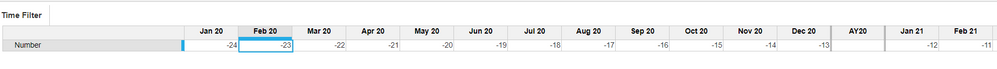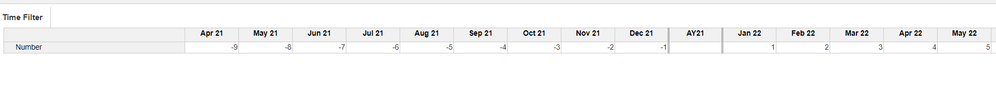Let me know if that works

Regards,

Anirudh

•Hi Elaine,

Assuming your line item is called Number, try this formula in the line item

`IF ITEM(Time) = Time.'Jan 19' THEN -12 ELSE PREVIOUS(Number) + 1`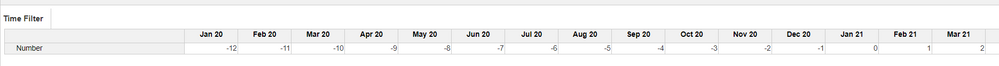Let me know if it works

•It looks good except that u hardcoded "-12" in the formula. Is there a way to not hard code it?

Assuming the parameters state that:

Start year: FY18

Launch year: FY20

That is where the users set the parameters. So the start month of Start year and Launch year is January. From that given info, how can i make the sequence without hardcording the distance between Jan 18 (being the -24) and Jan 20 (being the +1). No "0" in between the +1 and the -1 by the way.

Thanks again,

Elaine

•Here is solution that hopefully works for you, I broke it up into 3 line items:

Elaine: IF ITEM(Time) = IF ITEM(Time) = SYS Global.Elaine Date THEN 1 ELSE IF NEXT(Elaine) = 1 THEN -1 ELSE IF ITEM(Time) < SYS Global.Elaine Date THEN NEXT(Elaine) - 1 ELSE 0

Next Elaine: IF ITEM(Time) > SYS Global.Elaine Date THEN PREVIOUS(Elaine) + 1 ELSE 0

Final Elaine: Elaine + Next Elaine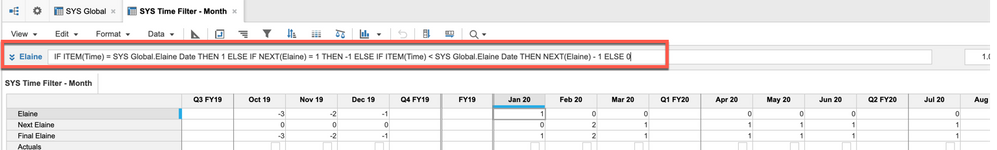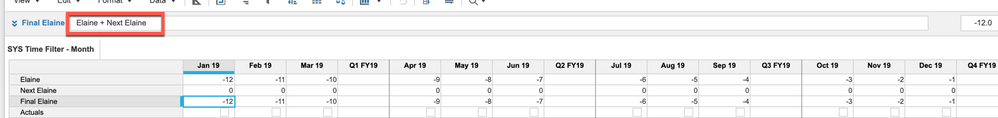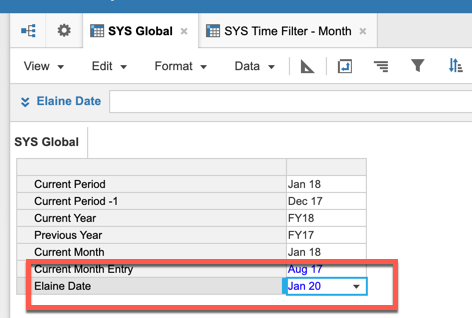Hope this helps,

Rob